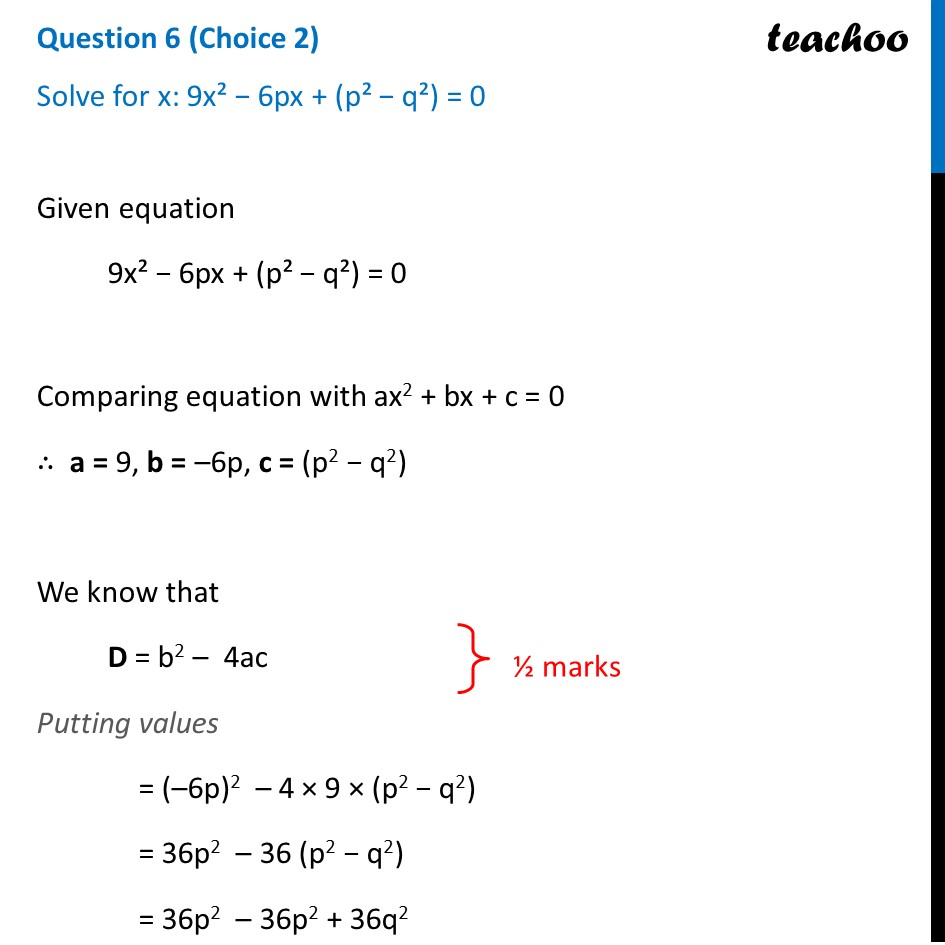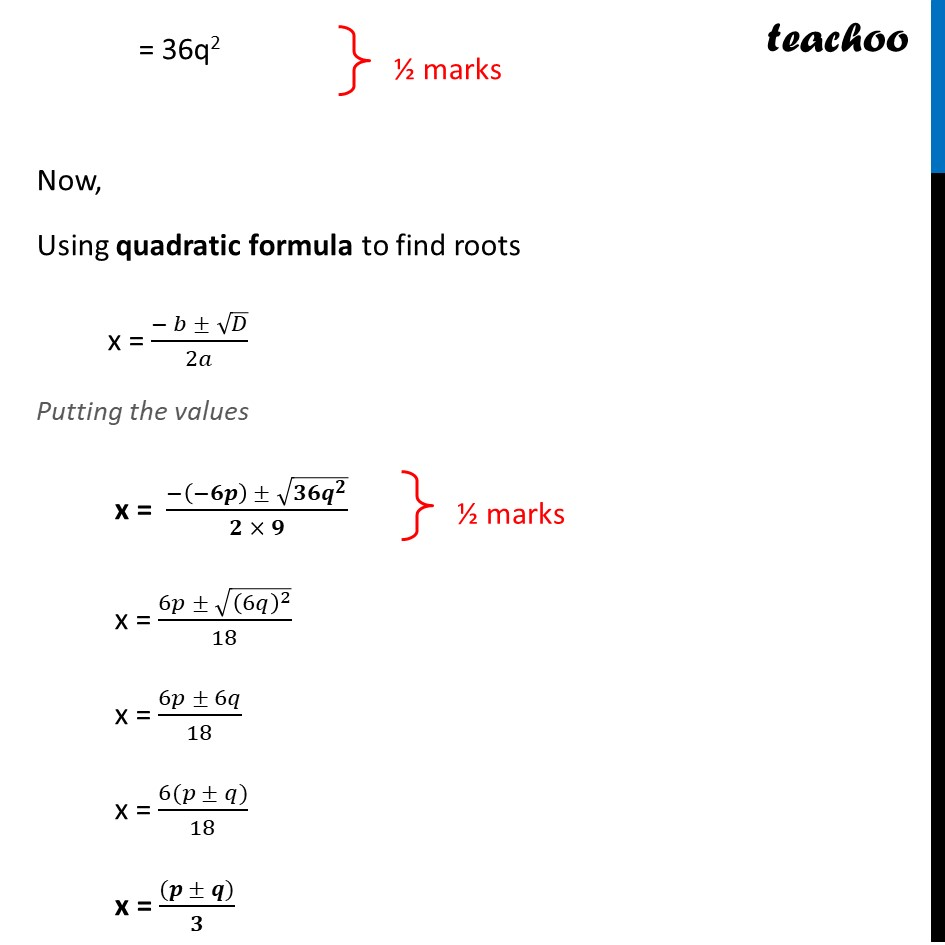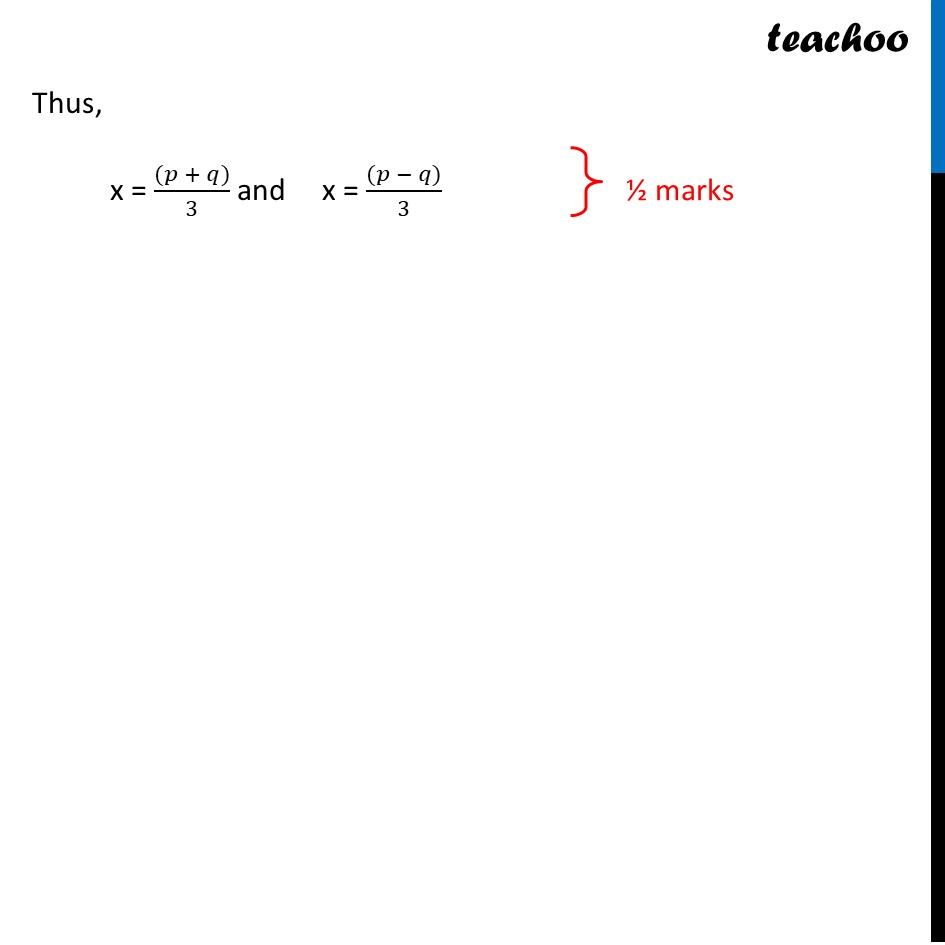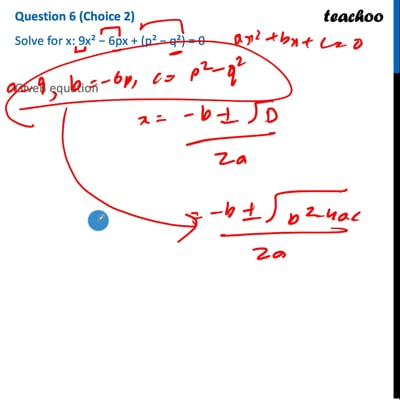CBSE Class 10 Sample Paper for 2022 Boards - Maths Standard [Term 2]

Class 10
Solutions of Sample Papers for Class 10 Boards

## Solve for x: 9x² - 6px + (p² - q²) = 0This video is only available for Teachoo black users

### Transcript

Solve for x: 9x² - 6px + (p² - q²) = 0 Given equation 9x² − 6px + (p² − q²) = 0 Comparing equation with ax2 + bx + c = 0 ∴ a = 9, b = –6p, c = (p2 − q2) We know that D = b2 – 4ac Putting values = (–6p)2 – 4 × 9 × (p2 − q2) = 36p2 – 36 (p2 − q2) = 36p2 – 36p2 + 36q2 = 36q2 Now, Using quadratic formula to find roots x = (− 𝑏 ± √𝐷)/2𝑎 Putting the values x = (−(−𝟔𝒑) ± √(𝟑𝟔𝒒^𝟐 ))/(𝟐 × 𝟗) x = (6𝑝 ± √((6𝑞)^2 ))/18 x = (6𝑝 ± 6𝑞)/18 x = (6(𝑝 ± 𝑞))/18 x = ((𝒑 ± 𝒒))/𝟑 Thus, x = ((𝑝 + 𝑞))/3 and x = ((𝑝 − 𝑞))/3